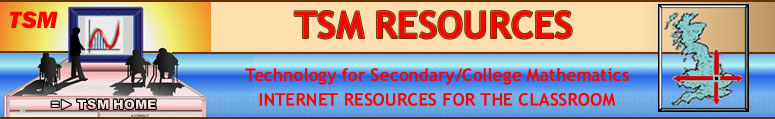TSM LONGER TUTORIALS Douglas Butler's YouTube Channel TSM Short Videos# AUTOGRAPH TUTORIALS by Douglas Butler

### Tutorial 1. Geometry and Measure (14 min)

A basic introduction
to how Autograph works.

The Whiteboard Mode
and multiple selection

dependent objects
Using the scribble tool

Reflection and rotation

Circles (coordinate geometry)
Circles (Euclidian geometry)

### Tutorial 2. Algebra and Graphing (20 min)

The Straight Line
Using the constant controller
The parabola y = x²; slow plot

Transformations of y = x²:
y = ax², y = (ax)²,
y = (x – a)² and y = x² + a
Fitting a parabola to an image
(human canon-ball)

Other topics to cover:
Linear Programming
The Autograph 'Extras' pages

### Tutorial 3. Advanced Topics (20 min)

Introduction to differentiation

Introducing trigonometry
Introducting integration

3D Autograph: conic sections
Volume of revolution of an area

Other topics to cover:
Vectors (2D and 3D)
Differential Eqns (1st/2nd order)
Parametric and Polar equations
(2D and 3D)

### Tutorial 4. Statistics and Handling Data (16 min)

Introduction to bivariate data
The principle of least squares
Pasting 2 columns of data

The Statistics page
Pasting a single column of data
The TSM Data resource
The dot plot

Grouping data for a histogram
Cumulative frequency diagram
Exporting a table of statistics
and a screen image

### Tutorial 5. Probability Distributions (13 min)

The binomial distribution
Fitting a dependent normal

Calculating binomial probabilities

The normal distribution
and calculations

Type 1 and Type 2 errors
The Central Limit Theorem

### Tutorial 6. Online Resources (7 min)

www.autograph-maths.com

The TSM Resources site
www.tsm-resources.com

including
TSM Data
and
TSM Images

### Tutorial 7. Onscreen Keyboard (9 min)

The onscreen keyboard allows users to control objects in Autograph, and to enter one-line mathematical expressions using a wide variety of symbols that are correctly interpreted, eg:

sin²2θ, 2x − 3y ≤ 6,
−b ± √(b²−4ac), y = |x|

It is also invaluable when using a whiteboard or a walk-about tablet. This tutorial also looks at changing languages

### => TSM Short Videos

Oundle, UK - March 2012